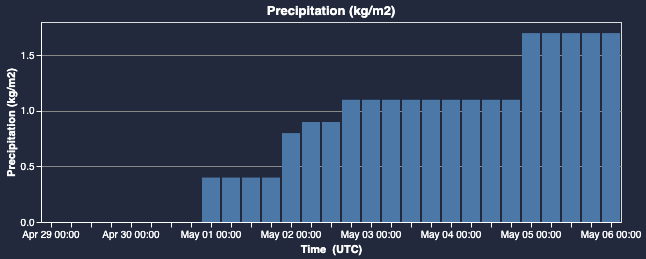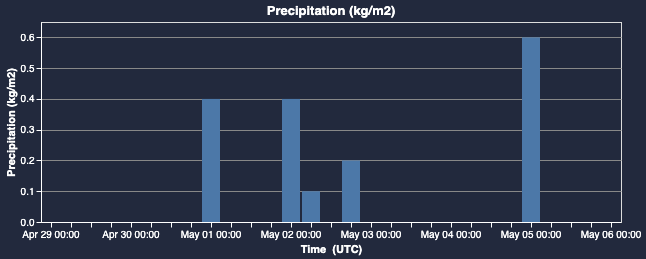1. Help Center
2. Spire Weather
3. Using the Spire Weather API

# Working with accumulated data values

## An overview of how to work with accumulated data values such as total precipitation.

Certain data variables returned by the Spire Weather API represent forecast-total accumulated values since the start of the forecast, rather than fixed interval accumulations such as 6 hour or 24 hour values.

Some notable examples of such variables are:

• Basic Bundle
• Total Precipitation
• Agricultural Bundle
• Surface Net Downward Shortwave Flux
• Surface Net Downward Longwave Flux
• Surface Net Upward Shortwave Flux
• Surface Net Upward Longwave Flux
• Net Upward Longwave Flux at Top of Atmosphere

Without any post-processing, the values from an accumulated variable would look like this:Over the course of the forecast's time-range, the values will only ever increase - since they are accumulating since the beginning of the forecast window.

With some simple post-processing on the data output, it is possible to get the difference between each value and the value that precedes it. Visualizing the result of this process would yield this:In both of these graph figures, the same exact data is being shown. They are simply 2 different methods of interpreting the data.

### Code Sample

Here is a code sample using the Weather Forecast Point API which demonstrates how a user could create 6-hour accumulations from the forecast-total value by differencing the totals from adjacent lead times.

For general information on types of precipitation, please see here.